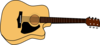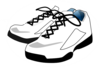877-601-6284
Get Started by Finding a Local Center

# Problem of the Week: October 19-23

Oct 23, 2020 | North AlpharettaLower Elementary:

Question:  Avery is making a fall scene collage out of paper shapes in art class. She cuts a piece of construction paper that is 10 inches long and 10 inches wide into 1–inch squares. Next, she cuts each square in half to make paper triangles. How many paper triangles does Avery have?

Solution:   If Avery cuts the paper into 1-inch strips, she will have 10 strips of paper. If she cuts each strip into 1-inch squares, she will have 10 for each strip. That means that she will have 10 × 10 = 100 squares. If she then cuts each square in half to make 2 triangles, she will have 100 × 2 = 200 paper triangles.

Upper Elementary:Question:  Twenty-four students split up into groups for music class. A third of the students are in the percussion group. Half of the students are in the choir group. The rest of the students are in the guitar group. How many students are there in the guitar group?

Solution:   One third of 24 is 24 ÷ 3 = 8 students, so there are 8 students in the percussion group. Half of 24 is 12, so there are 12 students in the choir group. That leaves 24 – 8 – 12 = 4 students in the guitar group.Middle School:

Question Paulina needs a new uniform for gym class. She buys a tee shirt that costs \$12.50, a pair of shorts that cost \$20.00, and a pair of sneakers that cost \$31.40. A 10% sales tax is applied to her purchase. How much money does Paulina spend on her new gym uniform?

Solution:   Before tax, the gym uniform costs \$12.50 + \$20.00 + 31.40 = \$63.90. To find the cost after tax, we multiply by 1.1; 1 for the price of the items, and an additional 0.1 for the tax. After tax, the uniform costs \$63.90 × 1.1 = \$70.29.

Algebra and Up:Question:  There are 125% as many students in sculpture class as there are in drawing class. If there are 15 more students in sculpture class than there are in drawing class, then how many students are in drawing class?